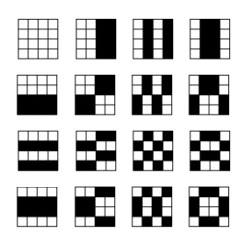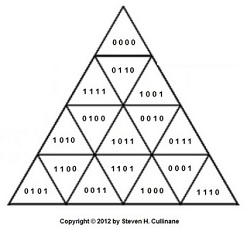Finite Geometry Notes   | Home | Site Map | Author |

Finite Relativity: The Triangular Version

(Continued from 1986)

 S. H. Cullinane  The relativity problem in finite geometry.  Feb. 20, 1986. This is the relativity problem: to fix objectively a class of equivalent coordinatizations and to ascertain the group of transformations S mediating between them. — H. Weyl, The Classical Groups , Princeton Univ. Pr., 1946, p. 16 In finite geometry "points" are often defined as ordered n-tuples of a finite (i.e., Galois) field GF(q). What geometric structures ("frames of reference," in Weyl's terms) are coordinatized by such n-tuples? Weyl's use of "objectively" seems to mean that such structures should have certain objective— i.e., purely geometric— properties invariant under each S. This note suggests such a frame of reference for the affine 4-space over GF(2), and a class of 322,560 equivalent coordinatizations of the frame. The frame: A 4×4 array. The invariant structure: The following set of 15 partitions of the frame into two 8-sets.A representative coordinatization:   0000  0001  0010  0011 0100  0101  0110  0111 1000  1001  1010  1011 1100  1101  1110  1111   The group: The group AGL(4,2) of 322,560 regular affine transformations of the ordered 4-tuples over GF(2). S. H. Cullinane  The relativity problem in finite geometry.  Nov. 22, 2012. This is the relativity problem: to fix objectively a class of equivalent coordinatizations and to ascertain the group of transformations S mediating between them. — H. Weyl, The Classical Groups , Princeton Univ. Pr., 1946, p. 16 In finite geometry "points" are often defined as ordered n-tuples of a finite (i.e., Galois) field GF(q). What geometric structures ("frames of reference," in Weyl's terms) are coordinatized by such n-tuples? Weyl's use of "objectively" seems to mean that such structures should have certain objective— i.e., purely geometric— properties invariant under each S. This note suggests such a frame of reference for the affine 4-space over GF(2), and a class of 322,560 equivalent coordinatizations of the frame. The frame: An array of 16 congruent equilateral subtriangles that make up a larger equilateral triangle. The invariant structure: The following set of 15 partitions of the frame into two 8-sets.A representative coordinatization:The group: The group AGL(4,2) of 322,560 regular affine transformations of the ordered 4-tuples over GF(2).

For some background on the triangular version,
see the Square-Triangle Theorem,
noting particularly the linked-to coordinatization picture.